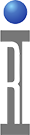This document lists the common RF measurements that can be performed by the CASSINI ATE and provides a brief description of each.

The measurements are categorized into 4 categories: VNA measurements, Testhead measurements, Receiver measurements, and Noise measurements.

VNA Measurements

The VNA measurements are all vector error corrected. Like the testhead, the VNA performs 8 measurements to get the 4 s-parameters. The VNA measurements assume the input and output frequencies to be the same, using the input frequency for the error correction.

VNA Measurements:ButtonUnitsCommentsS ParametersComplex VoltageMeasures all 4 s-parameters, vector error-corrected. Individual s-parameters must then be extracted using single-input calc buttons.S11 OnlyComplex VoltageMeasures S11 using reflection-only error correction. Full error correction could degrade accuracy if the other s-parameters are invalid, so this should be used when the other s-parameters are invalid, such as on one-port devices.S11Complex VoltageReturns S11. All 4 s-parameters are measured with full error correction. Then, only S11 is returned.Input VSWRN/AReturns S11 as a VSWR. All 4 s-parameters are measured with full error correction. Then, S11 is extracted and converted to VSWR.GainVoltsReturns the magnitude only for S21. All 4 s-parameters are measured with full error correction. Then, S21 is extracted and converted to voltage gain.PhaseDegreesReturns the phase only for S21. All 4 s-parameters are measured with full error correction. Then, S21 is extracted and converted to phase.

The testhead measurements are all based on the wave parameter measurements, taking 8 receiver voltage measurements to produce the requested result. If the testhead's s-parameter mode (SPAR MODE) is bidirectional, the measurements are taken in a traditional VNA method that reverses the signal through the DUT. In the unidirectional mode (recommended for most measurements), Roos' unique unidirectional process using a bi-state load is used. This process acquires all 8 measurements without reversing the signal through the DUT.

Some of the testhead buttons are very similar to VNA buttons. The main differences are: 1) In the testhead measurements the input and output frequencies are independently specified, whereas the VNA measurements (discussed later) assume the input and output frequencies to be the same. 2) Both measurements are vector error corrected, but the VNA measurements use more sophisticated error correction.ButtonUnitsCommentsWave Param at xxx Freq(1)N/AThe 'Wave Param at xxx Freq buttons are not exactly high-level measurement buttons. They produce raw measurements that can then be manipulated using calc buttons.Input PowerWattsMeasures power into the DUT with error correction at the input frequencyOutput PowerWattsMeasures power out of the DUT with error correction at the output frequencyConversion GainLinear Pwr RatioThe 'Conversion Gain' button measures the ratio of output power to input power.HarmonicsLinear Pwr RatioThe 'Harmonics' button measures the ratio of power at the receiver frequency to output power.

(1) xxx Freq is the input frequency, the output frequency, or the receiver frequency.
- For the input frequency, the system attempts to find the source that is being used based on the Testhead and Src12Output settings. (It does not look at the sources' power or on/off settings.) Whenever the result is ambiguous, it assumes source 1.
- For the output frequency, the system uses the 'FREQ REFERENCE', 'OUT FREQ OFFSET' and 'OUT FREQ SCALE' buttons from the 'System' panel.MeasurementDetectorP/L (2)Units (3)Typical UseCommentsVoltage (4)ComplexYComplex VoltageMeasure any synchronous signal. (All system components, except noise, are synchronized.)Use Voltage for most measurements except noise.Relative VoltageComplexNComplex VoltageOnly used for system service plans.Same as Voltage but without path loss correction.Power (4)ComplexYWattsMeasure non-synchronous signals.Relative PowerComplexNWattsOnly used for system service plans.Same as Power but without path loss correction.RMS PowerRMSYWattsScalar measurement of low level signals.No compensation for bandwidth.Noise PowerRMSYWatts/HzNoise close to the noise floor.Assumes 4 MHz IF BW (Not valid for 7 kHz BW) Compensates for BW. Corrects for system noise floorRelative Noise PwrRMSNWatts/HzOnly used for system service plans.Same as Noise Power but without path loss correction.Phase NoiseBoth(5)YWatts/HzPhase noise measurementsCompensates for bandwidth.